## Sunday, October 26, 2014

### Molecule counts in ChEMBL documents

I was curious about how many compounds are added to the data sources that are loaded into ChEMBL each year. This is an exploration around that theme, with a digression or two at the end, but no real conclusions

In :
```import time,random
import psycopg2
from __future__ import print_function
import pandas as pd
%pylab inline
%load_ext sql
```
```Populating the interactive namespace from numpy and matplotlib

```
```WARNING: pylab import has clobbered these variables: ['random']
`%matplotlib` prevents importing * from pylab and numpy

```

Start by pulling the number of new compounds appearing per year.

In :
```data = %sql postgresql://localhost/chembl_19 \
select miny as year,count(molregno) from \
(select molregno,min(year) as miny from compound_records join docs using (doc_id) where year>0 group by molregno) tmp \
group by miny order by miny desc;
df1 = data.DataFrame()
```
```37 rows affected.

```
In :
```df1.head(10)
```
Out:
year count
0 2014 15131
1 2013 70429
2 2012 69407
3 2011 67026
4 2010 84525
5 2009 65118
6 2008 61158
7 2007 52060
8 2006 33837
9 2005 30778

Obviously 2014 isn't complete yet, so we should ignore that one.

How many documents per year show up?

In :
```data = %sql \
select year,count(doc_id) as cnt from docs where year>0 \
group by year order by year desc ;
df2 = data.DataFrame()
```
```37 rows affected.

```
In :
```data=pd.merge(df1,df2)
data.rename(columns={'count':'molCount','cnt':'docCount'},inplace=True)
data.head(10)
```
Out:
year molCount docCount
0 2014 15131 895
1 2013 70429 4211
2 2012 69407 4174
3 2011 67026 4022
4 2010 84525 4599
5 2009 65118 4443
6 2008 61158 4063
7 2007 52060 3822
8 2006 33837 2132
9 2005 30778 2009

2010 was a big year.

Look at the general relationship between number of documents and number of compounds:

In :
```scatter(data['docCount'],data['molCount'])
_=xlabel('doc count')
_=ylabel('mol count')
```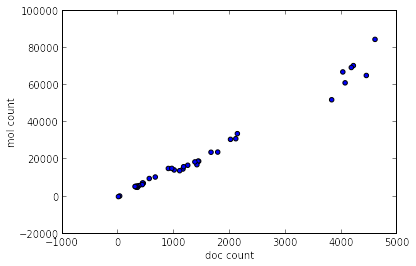That looks pretty linear; we can confirm that by looking at the ratio:

In :
```data['ratio']=data['molCount']/data['docCount']
```
In :
```scatter(data['year'],data['ratio'])
_=xlabel('year')
_=ylabel('mols / doc')
```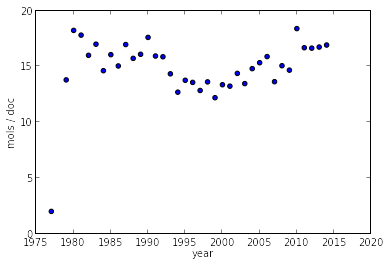There's a clear early outlier, leave it out:

In :
```data2=data[data.year>=1980]
scatter(data2['year'],data2['ratio'])
_=xlabel('year')
_=ylabel('mols / doc')
```
Out:
```<matplotlib.collections.PathCollection at 0x10b810950>
```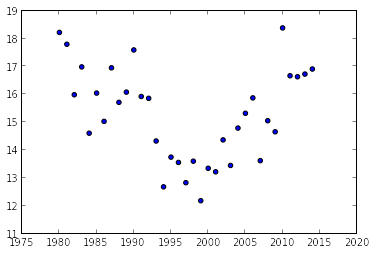Looks like there was dip in the late 90s. Odd.

# Compounds per paper

A more systematic look at the number of compounds appearing in each paper.

In :
```data = %sql postgresql://localhost/chembl_19 \
select count(molregno) numcmpds,doc_id from compound_records join docs using (doc_id) \
where doc_id>0 and year>1 group by doc_id
df1 = data.DataFrame()
```
```57115 rows affected.

```

Let's ignore documents that have more than 200 compounds; they probably aren't SAR papers anyway:

In :
```smaller=df1[df1.numcmpds<200]
print(len(smaller))
_=hist(smaller['numcmpds'],bins=20,log=True)
```
```57031

```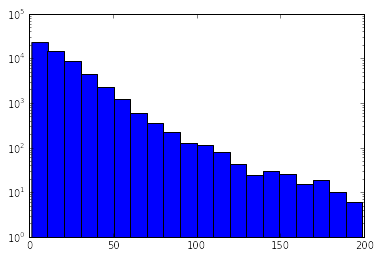# Papers per compound

Turn the question around and ask how many papers each compound appears in:

In :
```data = %sql postgresql://localhost/chembl_19 \
select count(doc_id) numdocs,molregno from compound_records join docs using (doc_id) \
where doc_id>0 and year>1 group by molregno order by numdocs desc
df1 = data.DataFrame()
```
```874168 rows affected.

```
In :
```_=hist(df1['numdocs'],bins=20,log=True)
_=xlabel('num papers')
```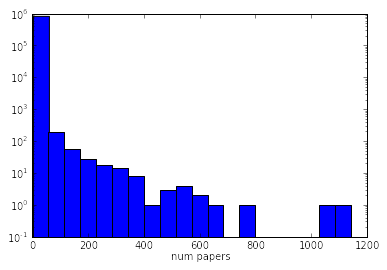In :
```df1.head()
```
Out:
numdocs molregno
0 1142 78759
1 1049 241
2 763 6579
3 667 8062
4 618 8873

## top 10 appearing molecules

In :
```data = %sql postgresql://localhost/chembl_19 \
select molregno,m as smiles,numdocs from ( \
select count(doc_id) numdocs,molregno from compound_records join docs using (doc_id) \
where doc_id>0 and year>1 group by molregno order by numdocs desc limit 10 \
) tmp join rdk.mols using (molregno)
df1 = data.DataFrame()
```
```10 rows affected.

```
In :
```from rdkit import Chem
from rdkit.Chem import Draw
from rdkit.Chem.Draw import IPythonConsole
from rdkit.Chem import PandasTools
```
In :
```PandasTools.AddMoleculeColumnToFrame(df1,smilesCol='smiles')
```
In :
```PandasTools.FrameToGridImage(df1,legendsCol='numdocs')
```
Out: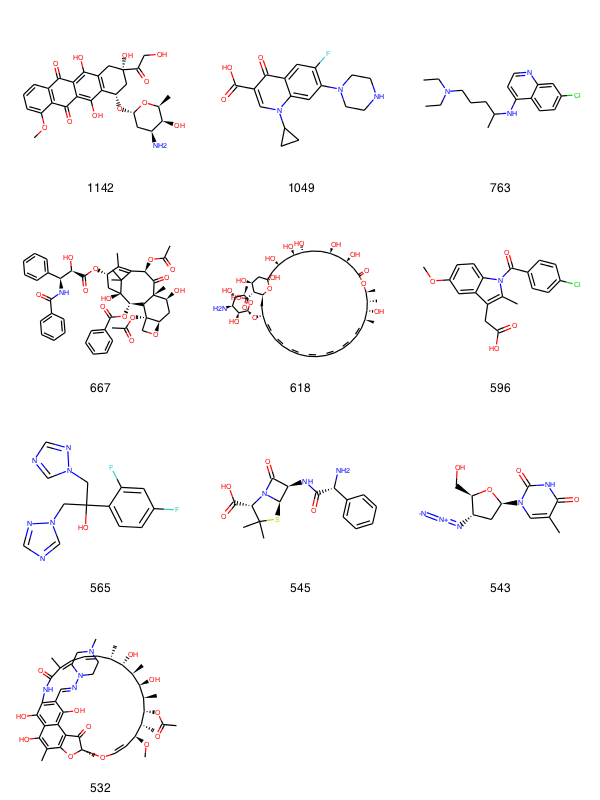In :
```df1
```
Out:
molregno smiles numdocs ROMol
0 78759 COc1cccc2c1C(=O)c1c(O)c3c(c(O)c1C2=O)C[C@@](O)(C(=O)CO)C[C@@H]3O[C@H]1C[C@H](N)[C@H](O)[C@H](C)O1 1142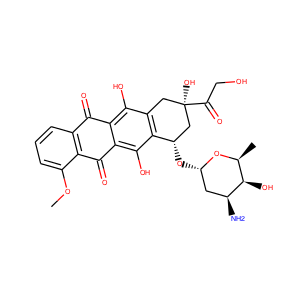1 241 O=C(O)c1cn(C2CC2)c2cc(N3CCNCC3)c(F)cc2c1=O 1049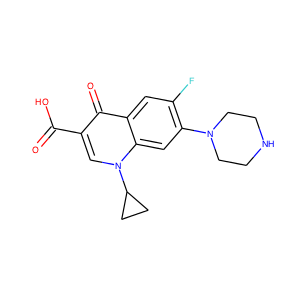2 6579 CCN(CC)CCCC(C)Nc1ccnc2cc(Cl)ccc21 763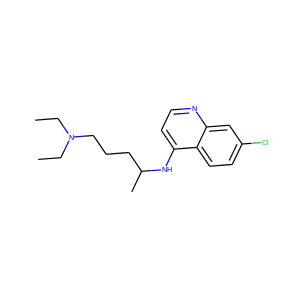3 8062 CC(=O)O[C@@H]1C2=C(C)[C@@H](OC(=O)[C@H](O)[C@@H](NC(=O)c3ccccc3)c3ccccc3)C[C@@](O)([C@@H](OC(=O)c3ccccc3)[C@@H]3[C@]4(OC(C)=O)CO[C@@H]4C[C@H](O)[C@@]3(C)C1=O)C2(C)C 667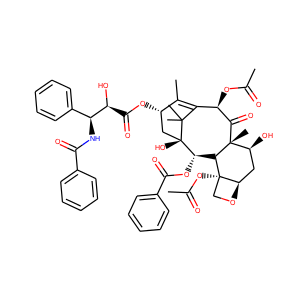4 8873 C[C@@H]1[C@H](O)[C@@H](C)C=CC=CC=CC=CC=CC=CC=C[C@H](O[C@@H]2O[C@H](C)[C@@H](O)[C@H](N)[C@@H]2O)C[C@@H]2O[C@](O)(C[C@@H](O)C[C@@H](O)[C@H](O)CC[C@@H](O)C[C@@H](O)CC(=O)O[C@H]1C)C[C@H](O)[C@H]2C(=O)O 618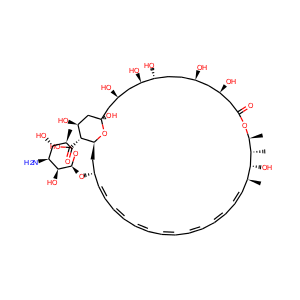5 173 COc1ccc2c(c1)c(CC(=O)O)c(C)n2C(=O)c1ccc(Cl)cc1 596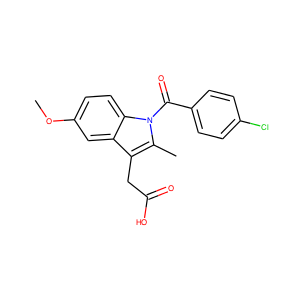6 13758 OC(Cn1cncn1)(Cn1cncn1)c1ccc(F)cc1F 565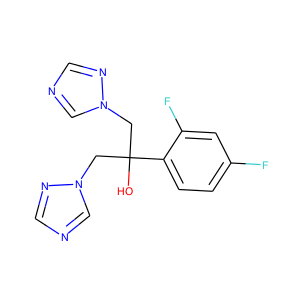7 305519 CC1(C)S[C@@H]2[C@H](NC(=O)[C@H](N)c3ccccc3)C(=O)N2[C@H]1C(=O)O 545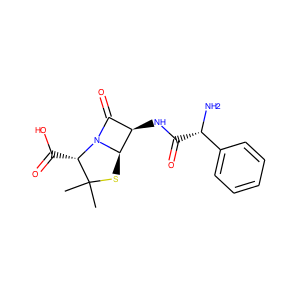8 27307 Cc1cn([C@H]2C[C@H](N=[N+]=[N-])[C@@H](CO)O2)c(=O)[nH]c1=O 543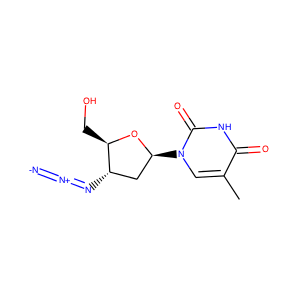9 365189 CO[C@H]1C=CO[C@@]2(C)Oc3c(c4c(O)c(/C=N/N5CCN(C)CC5)c(c(O)c4c(O)c3C)NC(=O)C(C)=CC=C[C@H](C)[C@H](O)[C@@H](C)[C@@H](O)[C@@H](C)[C@H](OC(C)=O)[C@@H]1C)C2=O 532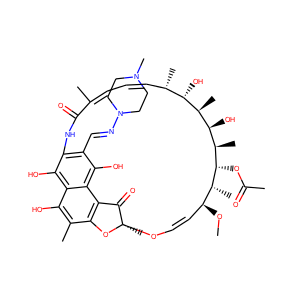Let's look some of these popular compounds up via the NCI Resolver. I would use ChemSpider for this, but their API is overly painful to use for these simple tasks.

In :
```import requests
def lookup(x):
resp = requests.get('http://cactus.nci.nih.gov/chemical/structure/'+x+'%09/names')
return '|'.join([x for x in resp.content.split('\n') if x.find('USAN')>-1 or x.find('USP')>-1])
df1['names']=df1['smiles'].map(lookup)
```
In :
```df1.drop('smiles',1)
```
Out:
molregno numdocs ROMol names
0 78759 1142Doxorubicin [USAN:BAN:INN]|Doxorubicin (USAN)
1 241 1049Ciprofloxacin (JAN/USP/INN)|Ciprofloxacin [USAN:BAN:INN]
2 6579 763Chloroquine (USP)|Chloroquine [USAN:BAN:INN]
3 8062 667Paclitaxel [USAN:BAN:INN]
4 8873 6185 173 596USAN|Indomethacin [USAN:BAN]|Indomethacin (USP)
6 13758 565Fluconazole (JAN/USAN/INN)|Fluconazole [USAN:BAN:INN:JAN]
7 305519 545Ampicillin (USP/INN)|Ampicillin [USAN:BAN:INN:JAN]
8 27307 543Zidovudine [USAN:BAN:INN:JAN]|Zidovudine (JAN/USP/INN)
9 365189 532I could look the missing two up in chemspider manually. Number 4 is Amphotericin B, and number 9 is Rifampicin. Definitely lots of antibiotics in that list.

In []:
```
```